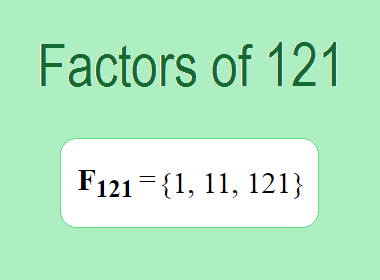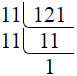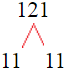# Factors of 121The factors of 121 are 1, 11, and 121 i.e. F121 = {1, 11, 121}. The factors of 121 are all the numbers that can divide 121 without leaving a remainder.

We can check if these numbers are factors of 121 by dividing 121 by each of them. If the result is a whole number, then the number is a factor of 121. Let's do this for each of the numbers listed above:

·        1 is a factor of 121 because 121 divided by 1 is 121.

·        11 is a factor of 121 because 121 divided by 11 is 11.

·        121 is a factor of 121 because 121 divided by 121 is 1.

## How to Find Factors of 121?

1 and the number itself are the factors of every number. So, 1 and 121 are two factors of 121. To find the other factors of 121, we can start by dividing 121 by the numbers between 1 and 121. If we divide 121 by 2, we get a remainder of 1. Therefore, 2 is not a factor of 121. If we divide 121 by 3, we get a remainder of 1. Therefore, 3 is also not a factor of 121.

Next, we can check if 4 is a factor of 121. If we divide 121 by 4, we get a remainder of 1. Therefore, 4 is also not a factor of 121. We can continue this process for all the possible factors of 121.

Through this process, we can find that the factors of 121 are 1, 11, and 121. These are the only numbers that can divide 121 without leaving a remainder.

********************

********************

## Properties of the Factors of 121

The factors of 121 have some interesting properties. One of the properties is that the sum of the factors of 121 is equal to 133. We can see this by adding all the factors of 121 together:

1 + 11 + 121 = 133

Another property of the factors of 121 is that they are all odd numbers. This is because 121 is an odd number, and any even number cannot divide an odd number without leaving a remainder.

Another property of the factors of 121 is that the prime factor of 121 is 11 only.

## Applications of the Factors of 121

The factors of 121 have several applications in mathematics. One of the applications is in finding the highest common factor (HCF) of two or more numbers. The HCF is the largest factor that two or more numbers have in common. For example, to find the HCF of 121 and 55, we need to find the factors of both numbers and identify the largest factor they have in common. The factors of 121 are 1, 11, and 121. The factors of 55 are 1, 5, 11, and 55. The largest factor that they have in common is 11. Therefore, the HCF of 121 and 55 is 11.

Another application of the factors of 121 is in prime factorization. Prime factorization is the process of expressing a number as the product of its prime factors. The prime factor of 121 11 only. We can express 121 as:

121 = 11 × 11

We can do prime factorization by division and factor tree method also. Here is the prime factorization of 121 by division method,121 = 11 × 11

Here is the prime factorization of 121 by the factor tree method,121 = 11 × 11

## Conclusion

The factors of 121 are the numbers that can divide 121 without leaving a remainder. The factors of 121 are 1, 11, and 121. The factors of 121 have some interesting properties, such as being odd numbers and having a sum of 133. The factors of 121 have several applications in mathematics, such as finding the highest common factor and prime factorization.